Learn to Use ITensor

main / tutorials / correlations

Calculating two-operator correlation functions

Thomas E. Baker—August 8, 2015

Let's calculate the two operator correlation function for a five site spin chain which is demonstrated in the coding recipe measuring a correlator for an MPS. The actual object we want to calculate is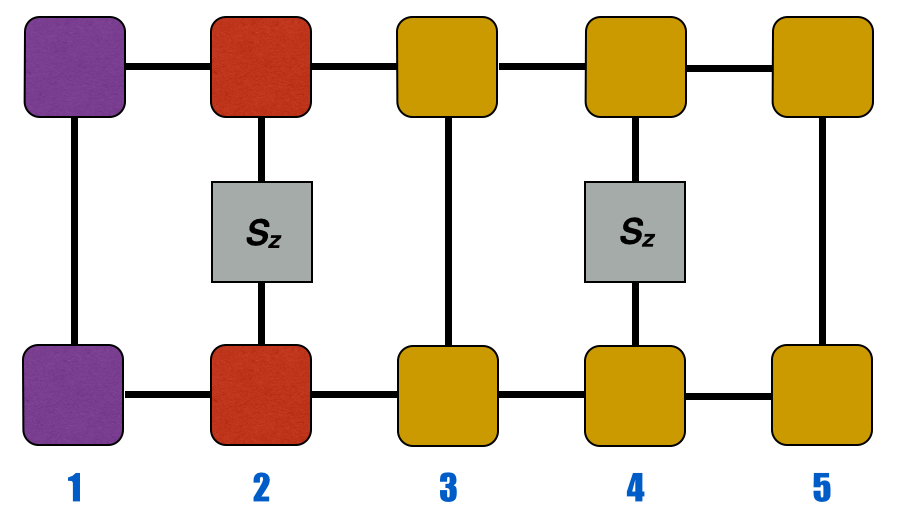$$=\langle\psi| S^z_2S^z_4|\psi\rangle$$

The ITensor code that produces this starts off by defining operators on the sites we want to measure. Here is some code used to generate the $S_z$ operator on sites 2 and 4: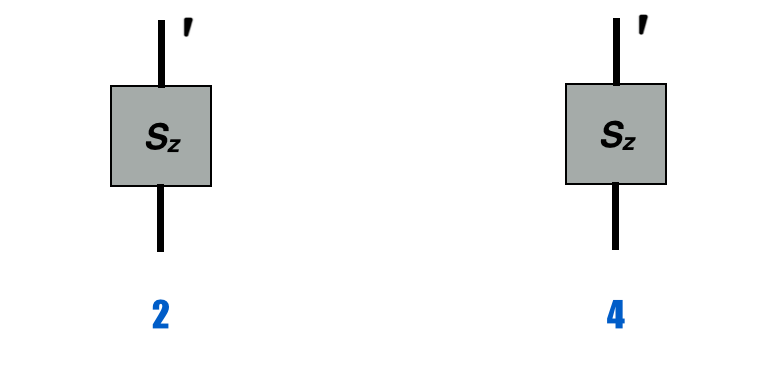auto Sz_2 = op(sites,"Sz",2);//automatically returns tensor on site
//2 with one prime and one unprimed index
auto Sz_4 = op(sites,"Sz",4);

The first thing to notice is that the orthogonality center (red) has been placed on site 2.

psi.position(2);

This allows for the left-normalized (purple) blocks of the MPS to give the identity on contraction, so we don't need to program anything special to deal with these. The same is true for the right-normalized (yellow) blocks outside the region we are interested in. Note that site 5 has this property. Technically, site 3 is also right normalized but it will not cancel to an identity since it lies in between two operators.

It is important to use the most efficient method of contracting the tensor network as we will do here; otherwise, the cost of computation will increase. We will contract all of the tensors one at a time into a tensor C which holds our partially completed diagram. The first tensor we will use to begin the sequence of contractions is the MPS tensor for site 2: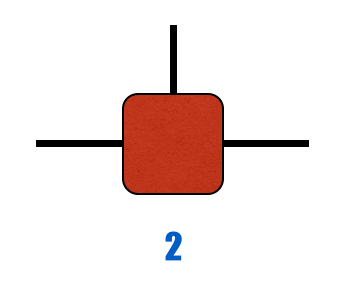ITensor C = psi(2);

This tensor has three indices. Two are links (horizontal lines) and the other is the physical index (vertical lines). This tensor must be multiplied by the operator we defined aboveC *= Sz_2;

The unprimed physical index of the $S_z$ operator is automatically contracted with the unprimed physical index of the MPS tensor. The dashed lines represent indices that ITensor will automatically contract when using *: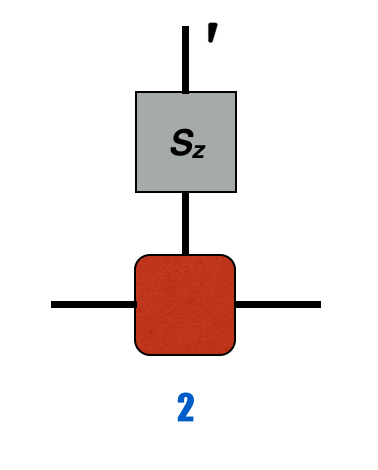Thus, we now have a primed physical index and unprimed link. We could have drawn the diagram as a new, single MPS site with a primed vertical index.

We need to apply the same tensor of the MPS for the bra vector, but this tensor must be conjugated and with a physical index that can match the primed index of the $S_z$ operator. Further, we must prime the link on the right; we do not want this line to contract with the link index on the ket. We want this index open to be contracted with another element in a subsequent step. If we only wanted to measure a single operator on one site, then we could leave this unprimed and the computation would be finished.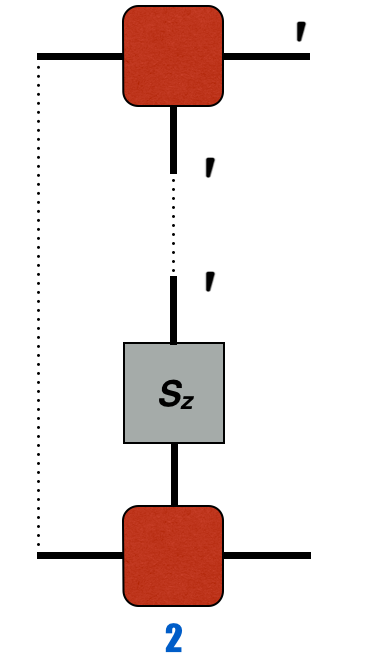auto ir = commonIndex(psi(2),psi(3),"Link"); //index to right of bra

C *= dag(prime(prime(psi(2),"Site"),ir)); //primes all Site type indices
//(physical indices) on the
//second site and index ir

Since we have gauged the MPS so it's orthogonality center is on site 2, the left-normalized (purple) blocks that we could draw here are equivalent to the identity. So, we do not need to include them in our code. Note that the primed link line (horizontal) will not contract with the primed physical index or the unprimed link index on the bottom. ITensor will not contract links and physical indices because they have different link identifications. The * operator only contracts the same index. The utility of calling a particular index a link or a site is to make it easy to prime all link or site indices on calling prime(psi,"Site") or similar for other uses.

The diagram so far looks like the following diagram and is stored in C.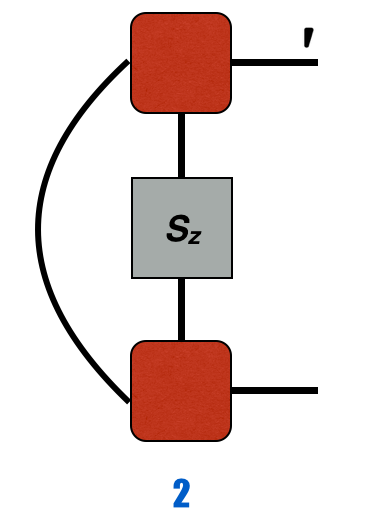Then, we contract with the next tensor in psi.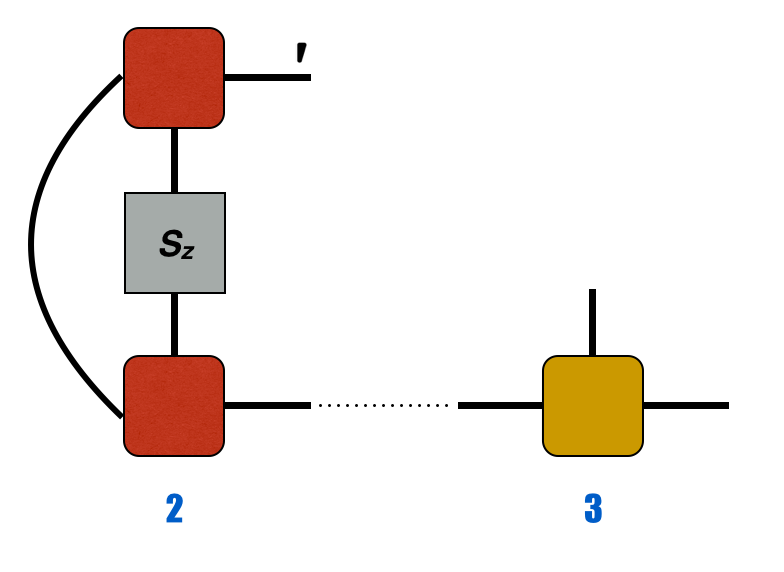C *= psi(3);

The equivalent element in the bra is next: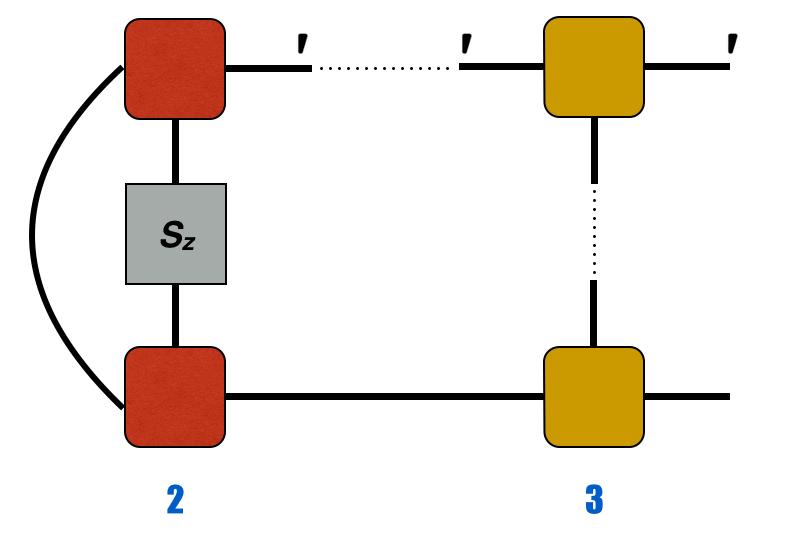Note that we do not prime the physical index here. We want the indices to match so that the vertical lines connect to the next piece we contract with. Rather, we simply want a primed link index for the same reasons as before.

Once again, we contract in the next part of the wavefunction:C *= psi(4);

Then we contract the second operator into the network: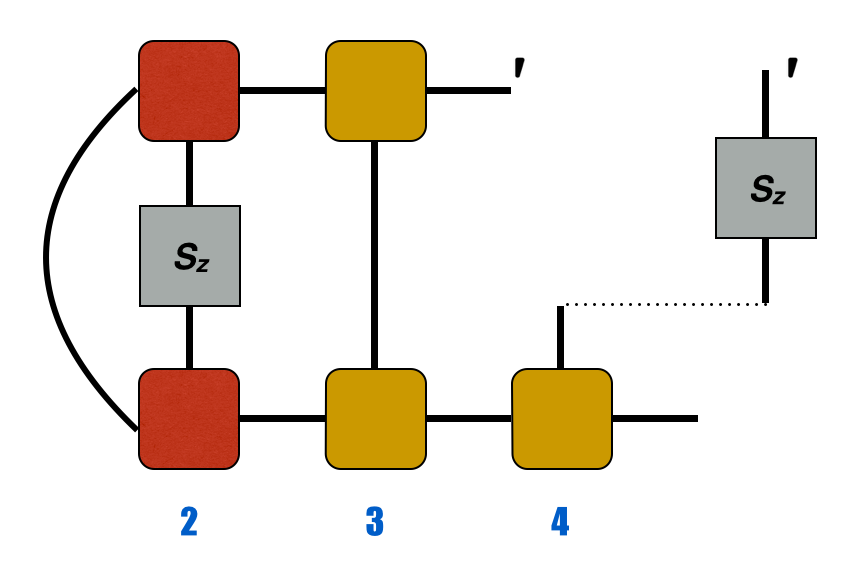C *=  Sz_4;

Then: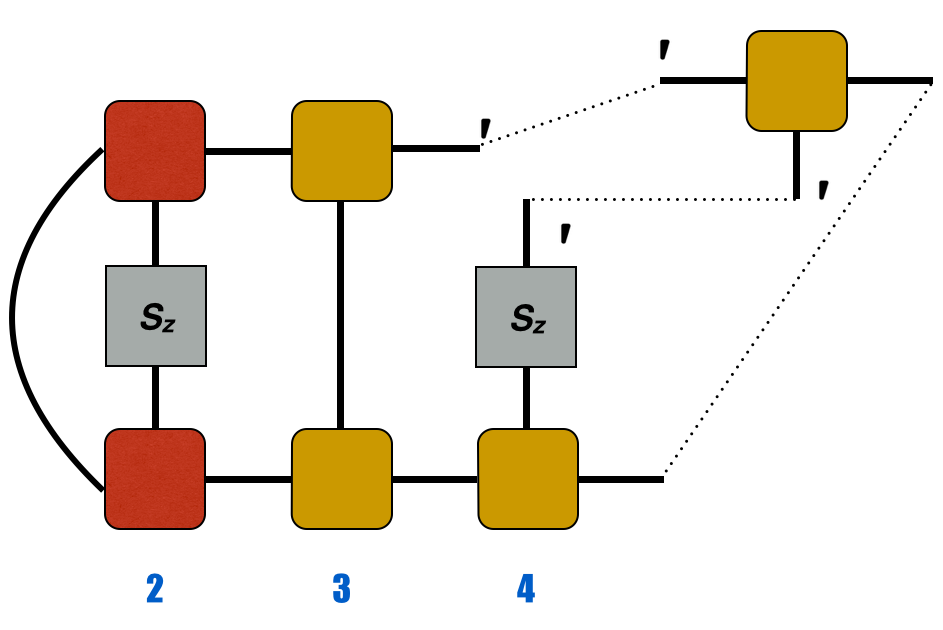C *=  dag(prime(prime(psi(4),"Site"),il));

This automatically gives the full correlation function after contracting the like indices.$$=\langle\psi|S_2^zS_4^z|\psi\rangle$$

This represents the entire tensor contraction to measure the correlation function. The final tensor C is a scalar (no indices); to extract its value as a real number we can use

elt(C);

Extending these ideas to more operators in a correlation function is straightforward. We add more operators to more sites just like those above.Back to TutorialsBack to Main